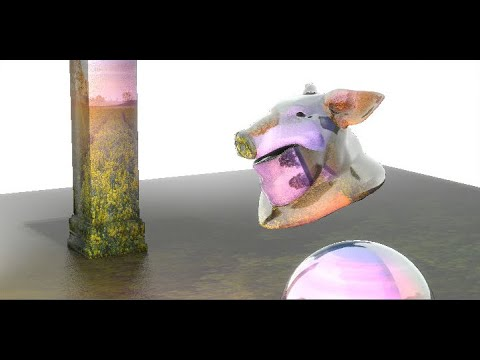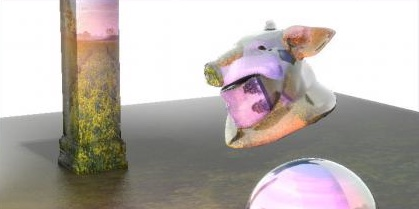# Custom Raytracer in COPs

calendar_today

21.04.2022

label

VEX, Rendering

mouse

Houdini 17.0

### 1 Introduction

Let's dissect a custom Raytracer written in VEX. It runs in COPs and supports:
- Meshes with UV coordinates
- Multiple point lights (including color, intensity, size)
- Area shadows and light attenuation
- Ambient occlusion
- Specular highlights
- Reflections with varying roughness

### 2 Camera

In the compositing context (COP) there are some global variables such as XRES and YRES storing the resolution of our image and X and Y for the normalized screen coordinate ranging from 0.0 to 1.0. After calculating the aspect ratio `YRES / float(XRES)` and centering our canvas `set(X - 0.5, (Y - 0.5)` we access the camera matrix `optransform(cam)` and position `cracktransform(0,0,0,0,0, xform_cam)`.

``````// CAMERA
float aspect_cam = YRES / float(XRES);
vector pos_canvas = set(X - 0.5, (Y - 0.5) * aspect_cam, 0.0) * 0.036;
matrix xform_cam = optransform(cam);
vector pos_cam = cracktransform(0,0,0,0,0, xform_cam);``````

By multiplying the canvas position with the camera transformation matrix we are transforming the canvas to the orientation of the camera. We put the focal point a little behind the sensor to create a camera frustum and create ray directions with a certain range.

``````vector pos_sensor = pos_canvas * xform_cam;
vector pos_focal = set(0.0, 0.0, focal) * xform_cam;
vector dir_sensor = normalize(pos_sensor - pos_focal);
vector ray_sensor = dir_sensor * vector(range_cam);``````

### 3 Texture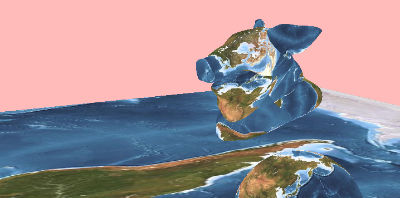We use the `intersect` function to shoot rays towards our geometry and collect the primitive `prim_hit`, the UV position on the primitive `st_hit` and the world position `pos_hit` we have hit.

primuv uses the primitive number and the primitives intrinsic UV position to get the texture UV coordinate stored as `uvw_hit`. Next we gather the texture path string using the `prim` function. Lastly we sample the texture pixels from a `colormap`.

``````// TEXTURE
vector pos_hit;
vector st_hit;
int prim_hit = intersect(geo, pos_sensor, ray_sensor, pos_hit, st_hit);
vector uvw_hit = primuv(geo, 'uv', prim_hit, st_hit);
string tex_diff = prim(geo, 'tex_diff', prim_hit);
vector map_diff = colormap(tex_diff, uvw_hit);``````

### 4 Occlusion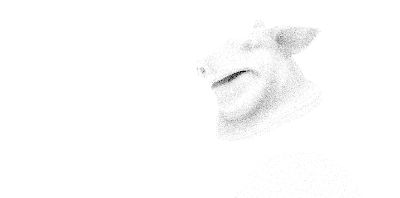Optionally we can calculate the occlusion around the shading location. Based on the primitive number and primitives UV information, we request the surface normal at the given point of our mesh and slightly `1e-5` add it to the world position. After defining an appropriate angle for our occlusion rays, we sum up the number of times our occlusion rays have hit other polygons surrounding our hit location. For normalizing the occlusion value we divide the number of hits by the number of rays we have sent. The result is a greyscale map that will add a darker shade to occluded areas.

``````// OCCLUSION
vector nml_hit = primuv(geo, 'N', prim_hit, st_hit);
vector pos_hit_ray = pos_hit + nml_hit * 1e-5;
float angle_occ = 0.5 * M_PI;
int sum_occ = 0;
for(int i = 0; i < samples_occ; i++){
vector2 u_occ = rand(pos_canvas + vector(i + i@Frame));
vector ray_occ = sample_direction_cone(nml_hit, angle_occ, u_occ) * dist_occ;
vector pos_hit_occ;
vector st_hit_occ;
int prim_hit_occ = intersect(geo, pos_hit_ray, ray_occ, pos_hit_occ, st_hit_occ);
sum_occ += prim_hit_occ < 0;
}
float occ = sum_occ / float(samples_occ);``````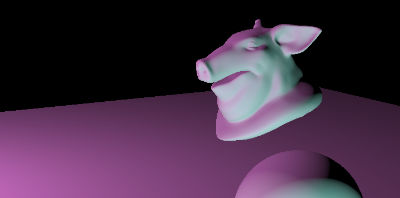Next, we calculate how much light the surfaces reflect. For this we first sample the `rough`ness of the material and sum up the amount of light coming from our point light sources. Our lights point cloud contains attributes for position, color, intensity and the area size. We integrate the quadratic attenuation into the distance calculation, use the `dot` product to consider the angle of the surfaces towards the light sources and `clamp` negative values.

``````// SHADING
float rough_hit = primuv(geo, 'rough', prim_hit, st_hit);
float bright = 0.0;
float spec = 0.0;
int num_lights = npoints(lights);
for(int i = 0; i < num_lights; i++){
vector pos_light = point(lights, 'P', i);
vector clr_light = point(lights, 'Cd', i);
float intens_light = point(lights, 'intens', i);
float area_light = point(lights, 'area', i);
vector dir_light = normalize(pos_light - pos_hit);

float dist_light = distance(pos_light, pos_hit);
float atten = intens_light / (dist_light * dist_light);
shading += clamp( dot(dir_light, nml_hit), 0.0, 1.0) * clr_light * atten;``````

### 6 Specular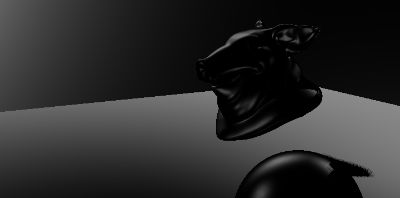To add specular highlights we will reflect the direction from the sensor towards the scene against the surface normal `reflect(dirsensor, nml_hit)` and compare the direction of the specular direction against the direction of the light `dot(dir_spec, dir_light)`. After sharpening the highlight with a power function `pow(angle_spec, 50.0)`, we simply add it to the specular amount after multiplying it with the complemented roughness `(1 - rough_hit)` of the underlying material.

``````// SPECULAR
vector dir_spec = reflect(dir_sensor, nml_hit);
float angle_spec = dot(dir_spec, dir_light);
float angle_spec_mod = pow(angle_spec, 50.0);
spec += angle_spec_mod * (1 - rough_hit);``````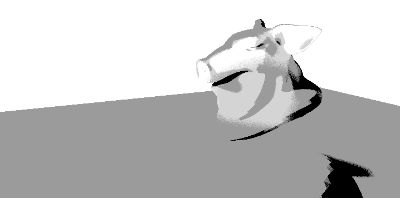The shadow calculation is done by shooting rays from the surface location towards each light source. Rays not hitting anything are added to the `sum_bright` which is divided by the number of rays `sum_bright / float(samples_shadow)`.

``````// SHADOWS
int sum_bright = 0;
for(int j = 0; j < samples_shadow; j++){
vector2 u_shadow = rand(pos_canvas + vector(j + i@Frame));
}
}
bright /= num_lights;``````

### 8 Reflection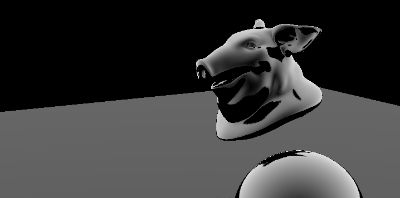The reflection (in our case its just the reflection amount to quickly fake it) is calculated similarily to the specular highlights. However, integrating a (also fake) fresnel term helps fading out the reflections when looking at the surface from a flat angle.

Also, depending on the gathered roughness we send out a wide or narrow cone of rays to find out whether at this location our rays hit surrounding polygons or not. Not hitting anythin will result in adding a bright reflection on top of our shading.

``````// REFLECTION
float amount_refl = primuv(geo, 'amount_refl', prim_hit, st_hit);
int sum_refl = 0;
vector dir_refl = reflect(dir_sensor, nml_hit);
float fresnel = 1.0 - dot(dir_sensor * vector(-1.0), nml_hit);

for(int i = 0; i < samples_refl; i++){
vector2 u_refl = rand(pos_canvas + vector(i + i@Frame));
vector ray_refl = sample_direction_cone(dir_refl, rough_hit, u_refl) * 100;
vector pos_hit_refl;
vector st_hit_refl;
int prim_hit_refl = intersect(geo, pos_hit_ray, ray_refl, pos_hit_refl, st_hit_refl);
sum_refl += prim_hit_refl < 0;
}
float refl = amount_refl * fresnel * (sum_refl / float(samples_refl));
float spec_fresnel = spec * fresnel * bright;``````

### 9 OutputIn the output section we add and multiply all passes such as diffuse surface color, diffuse shading, brightness, occlusion, reflections and specular hightlights. Where the sensor rays have not hit any geometry we will use a sky color. The combined color vector is then assigned to the color components R, G and B of the image plane. In another wrangle we also add a blurred layer with bright parts of our rendered image to overlay a glow effect.

``````// OUTPUT
vector sky = vector(1.0);
vector comp = map_diff * shading * bright * occ + refl + spec_fresnel;
vector color = prim_hit < 0 ? sky : comp;
vector color_clamp = clamp(color, vector(0.0), vector(1.0));

assign(R, G, B, color_clamp);``````

### 10 Full code

Here is the full code. Please keep in mind its experimental and contains lots of shortcuts and simplifications.

``````// CAMERA
float aspect_cam = YRES / float(XRES);
vector pos_canvas = set(X - 0.5, (Y - 0.5) * aspect_cam, 0.0) * 0.036;
matrix xform_cam = optransform(cam);
vector pos_cam = cracktransform(0,0,0,0,0, xform_cam);

vector pos_sensor = pos_canvas * xform_cam;
vector pos_focal = set(0.0, 0.0, focal) * xform_cam;
vector dir_sensor = normalize(pos_sensor - pos_focal);
vector ray_sensor = dir_sensor * vector(range_cam);

// TEXTURE
vector pos_hit;
vector st_hit;
int prim_hit = intersect(geo, pos_sensor, ray_sensor, pos_hit, st_hit);
vector uvw_hit = primuv(geo, 'uv', prim_hit, st_hit);
string tex_diff = prim(geo, 'tex_diff', prim_hit);
vector map_diff = colormap(tex_diff, uvw_hit);

// OCCLUSION
vector nml_hit = primuv(geo, 'N', prim_hit, st_hit);
vector pos_hit_ray = pos_hit + nml_hit * 1e-5;
float angle_occ = 0.5 * M_PI;
int sum_occ = 0;
for(int i = 0; i < samples_occ; i++){
vector2 u_occ = rand(pos_canvas + vector(i + i@Frame));
vector ray_occ = sample_direction_cone(nml_hit, angle_occ, u_occ) * dist_occ;
vector pos_hit_occ;
vector st_hit_occ;
int prim_hit_occ = intersect(geo, pos_hit_ray, ray_occ, pos_hit_occ, st_hit_occ);
sum_occ += prim_hit_occ < 0;
}
float occ = sum_occ / float(samples_occ);

float rough_hit = primuv(geo, 'rough', prim_hit, st_hit);
float bright = 0.0;
float spec = 0.0;
int num_lights = npoints(lights);
for(int i = 0; i < num_lights; i++){
vector pos_light = point(lights, 'P', i);
vector clr_light = point(lights, 'Cd', i);
float intens_light = point(lights, 'intens', i);
float area_light = point(lights, 'area', i);
vector dir_light = normalize(pos_light - pos_hit);

float dist_light = distance(pos_light, pos_hit);
float atten = intens_light / (dist_light * dist_light);
shading += clamp( dot(dir_light, nml_hit), 0.0, 1.0) * clr_light * atten;

// SPECULAR
vector dir_spec = reflect(dir_sensor, nml_hit);
float angle_spec = dot(dir_spec, dir_light);
float angle_spec_mod = pow(angle_spec, 50.0);
spec += angle_spec_mod * (1 - rough_hit);

int sum_bright = 0;
for(int j = 0; j < samples_shadow; j++){
vector2 u_shadow = rand(pos_canvas + vector(j + i@Frame));
}
}
bright /= num_lights;

// REFLECTION
float amount_refl = primuv(geo, 'amount_refl', prim_hit, st_hit);
int sum_refl = 0;
vector dir_refl = reflect(dir_sensor, nml_hit);
float fresnel = 1.0 - dot(dir_sensor * vector(-1.0), nml_hit);

for(int i = 0; i < samples_refl; i++){
vector2 u_refl = rand(pos_canvas + vector(i + i@Frame));
vector ray_refl = sample_direction_cone(dir_refl, rough_hit, u_refl) * 100;
vector pos_hit_refl;
vector st_hit_refl;
int prim_hit_refl = intersect(geo, pos_hit_ray, ray_refl, pos_hit_refl, st_hit_refl);
sum_refl += prim_hit_refl < 0;
}
float refl = amount_refl * fresnel * (sum_refl / float(samples_refl));
float spec_fresnel = spec * fresnel * bright;

// OUTPUT
vector sky = vector(1.0);
vector comp = map_diff * shading * bright * occ + refl + spec_fresnel;
vector color = prim_hit < 0 ? sky : comp;
vector color_clamp = clamp(color, vector(0.0), vector(1.0));

assign(R, G, B, color_clamp);``````